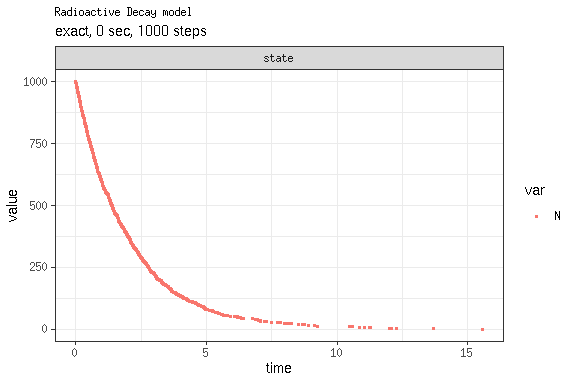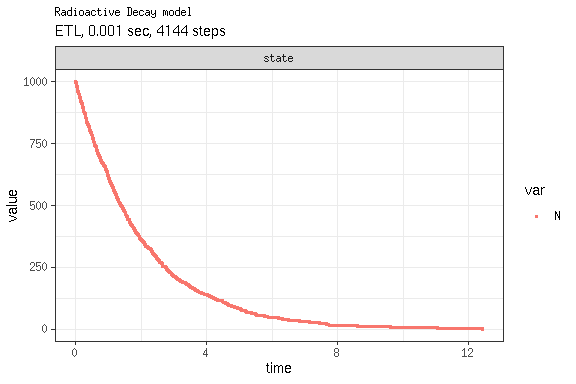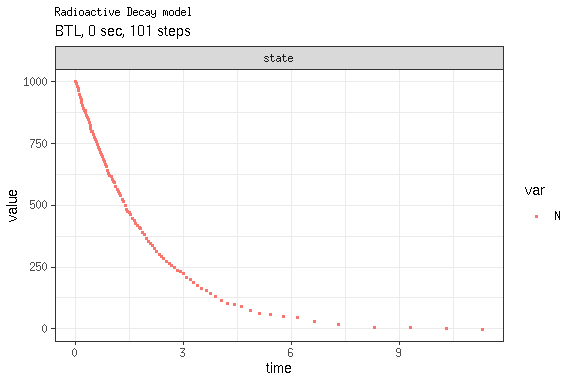# Radioactive Decay model (Gillespie, 1977)

This model is also known as the irreversible isomerization reaction set. It consists of a single species and single reaction channels,

X --c--> 0

Define parameters

library(GillespieSSA2)
params <- c(k = 0.5)
final_time <- 20
initial_state <- c(N = 1000)

Define reactions

reactions <- list(
reaction("k * N", c(N = -1))
)

Run simulations with the Exact method

set.seed(1)
out <- ssa(
initial_state = initial_state,
reactions = reactions,
params = params,
final_time = final_time,
method = ssa_exact(),
sim_name = sim_name
)
plot_ssa(out)Run simulations with the Explict tau-leap method

set.seed(1)
out <- ssa(
initial_state = initial_state,
reactions = reactions,
params = params,
final_time = final_time,
method = ssa_etl(tau = .003),
sim_name = sim_name
)
plot_ssa(out)Run simulations with the Binomial tau-leap method

set.seed(1)
out <- ssa(
initial_state = initial_state,
reactions = reactions,
params = params,
final_time = final_time,
method = ssa_btl(),
sim_name = sim_name
)
plot_ssa(out)